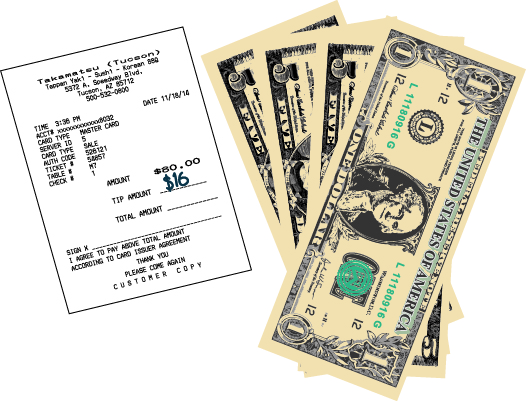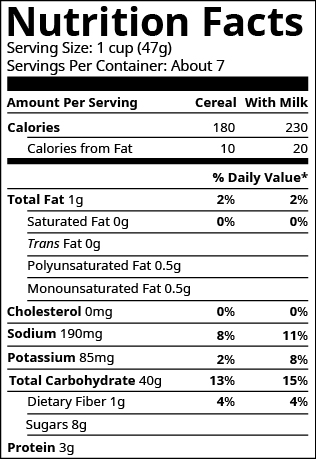## Solving Problems Using Percents

### Learning Outcome

• Evaluate expressions and word problems involving percents

In this section we will solve percent questions by identifying the parts of the problem. We’ll look at a common application of percent—tips to a server at a restaurant—to see how to set up a basic percent application.

When Aolani and her friends ate dinner at a restaurant, the bill came to $\text{\80}$. They wanted to leave a $20\%$ tip. What amount would the tip be?

To solve this, we want to find what amount is $20\%$ of $\80$. The $\80$ is called the base. The percent is the given $20\%$. The amount of the tip would be $0.20(80)$, or $\16$ — see the image below. To find the amount of the tip, we multiplied the percent by the base.

A $20\%$ tip for an $\80$ restaurant bill comes out to $\16$.

##### Pieces of a Percent Problem

Percent problems involve three quantities:  the base amount (the whole), the percent, and the amount (a part of the whole or partial amount).

The amount is a percent of the base.

Let’s look at another example:

Jeff has a Guitar Strings coupon for $15\%$ off any purchase of $100$ or more. He wants to buy a used guitar that has a price tag of $220$ on it. Jeff wonders how much money the coupon will take off the original $220$ price. Problems involving percents will have some combination of these three quantities to work with: the percent, the amount, and the base. The percent has the percent symbol (%) or the word percent. In the problem above, $15\%$ is the percent off the purchase price. The base is the whole amount or original amount. In the problem above, the “whole” price of the guitar is $220$, which is the base. The amount is the unknown and what we will need to calculate.

There are thee cases: a missing amount, a missing percent or a missing base. Let’s take a look at each possibility.

## Solving for the Amount

When solving for the amount in a percent problem, you will multiply the percent (as a decimal or fraction) by the base. Typically we choose the decimal value for percent.

$\text{percent}\cdot{\text{base}}=\text{amount}$

### Example

Find $50\%$ of $20$

Solution:

First identify each piece of the problem:

percent: $50\%$ or $.5$

base: $20$

amount: unknown

Now plug them into your equation $\text{percent}\cdot{\text{base}}=\text{amount}$

$.5\cdot{20}= ?$

$.5\cdot{20}= 10$

Therefore, $10$ is the amount or part that is $50\%$ of $20$.

### Example

What is $25\%$ of $80$?

## Solving for the Percent

When solving for the percent in a percent problem, you will divide the amount by the base. The equation above is rearranged and the percent will come back as a decimal of fraction you can report in the form asked of you.

$\Large{\frac{\text{amount}}{\text{base}}}\normalsize=\text{percent}$

### Example

What percent of $320$ is $80$?

Solution:

First identify each piece of the problem:

percent: unknown

base: $320$

amount: $80$

Now plug the values into your equation $\Large{\frac{\text{amount}}{\text{base}}}\normalsize=\text{percent}$

$\large\frac{80}{320}\normalsize=?$

$\large\frac{80}{320}\normalsize=.25$

Therefore, $80$ is $25\%$ of $320$.

## Solving for the Base

When solving for the base in a percent problem, you will divide the amount by the percent (as a decimal or fraction). The equation above is rearranged and you will find the base after plugging in the values.

$\Large{\frac{\text{amount}}{\text{percent}}}\normalsize=\text{base}$

### EXample

$60$ is $40\%$ of what number?

Solution:

First identify each piece of the problem:

percent:$40\%$ or $.4$

base: unknown

amount: $60$

Now plug the values into your equation $\Large{\frac{\text{amount}}{\text{percent}}}\normalsize=\text{base}$

$(60)\div(.4)=?$

$(60)\div(.4)=150$

Therefore, $60$ is $40\%$ of $150$.

### Example

An article says that $15\%$ of a non-profit’s donations, about $30,000$ a year, comes from individual donors.  What is the total amount of donations the non-profit receives?

### TRY IT

Here are a few more percent problems for you to try.

### try it

Many applications of percent occur in our daily lives, such as tips, sales tax, discount, and interest. To solve these applications we’ll translate to a basic percent equation, just like those we solved in the previous examples in this section. Once you translate the sentence into a percent equation, you know how to solve it.

### example

Dezohn and his girlfriend enjoyed a dinner at a restaurant, and the bill was $\text{\68.50}$. They want to leave an $\text{18%}$ tip. If the tip will be $\text{18%}$ of the total bill, how much should the tip be?

Solution

 What are you asked to find? the amount of the tip What formula/equation should you use? $\text{percent}\cdot{\text{base}}=\text{amount}$ Substitute in the correct values. $(.18)\cdot{68.50}$ Solve. $(.18)\cdot{68.50}=12.33$ Write a complete sentence that answers the question. The couple should leave a tip of $\text{\12.33}$.

### try it

In the next video we show another example of finding how much tip to give based on percent.

### example

The label on Masao’s breakfast cereal said that one serving of cereal provides $85$ milligrams (mg) of potassium, which is $\text{2%}$ of the recommended daily amount. What is the total recommended daily amount of potassium?## Contribute!

Did you have an idea for improving this content? We’d love your input.